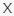# Video Math Quiz VideoQuizStar

Try: Video Math Quiz VideoQuizHero

Q 1. Let’s start with an easy one. Click PLAY to watch the video below and see if you can answer the question.

Choices:

A – 8%
B – 20%
C – 25%
D – 33%

B – 20%

Try: Video Math Quiz VideoQuizHero

Q 2. Watch the video below and try to answer this question about geometry! (Have a look at this triangle!)

Choices:

5
6
7
12

5

Q 3. Pay close attention to the video below. Can you answer the question? (How many spheres were there?)

Choices:

A – 156
B – 158
C – 161
D – 164
E – 166
F – 169

C – 161

Try Version2: Video Math Quiz VideoQuizStar 2

Q 4. Don’t lose focus on this one. Can you solve the equation in the video? (Solve the equation)

Choices:

A – 0.0625
B – 0.25
C – 13
D – 17.5
E – 52
F – 70
G – 94
H – 367

E – 52

Try Version2: Video Math Quiz VideoQuizStar 2

Q 5. Let’s test your logic skills! Watch the video and try to find the correct answer! (Determine the value of each object to solve the equation)

Choices:

A
B
C
D
E
F

B

Try: Video Math Quiz VideoQuizHero

### 3 thoughts on “Video Math Quiz VideoQuizStar”

•April 30, 2020 at 11:52

I had 6 questions in my quiz, number 4 was not in your list. The question was:
‘Can you solve this tricky equation? ‘

🔘×⚫=◼
🔘+⚫=◼-

A: 3
B: 8
C: 12
D: 18

I randomly chose B and luckily got it right but it was pure chance.

•